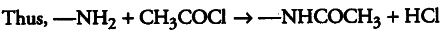# Find the number of amino groups present per molecule

A compound with molecular mass 180u is acylated with \$C{ H }_{ 3 }COCL\$, to get a compound with molecular mass 390 u. Find the number of amino groups present per molecule of the former compound.

During acylation, one H-atom with atomic mass 1 amu of —\$N{ H }{ 2 }\$ group is replaced by an acetyl group CO—CH3 [molecular mass = 43 u].The above equation suggests that the acylation of each —\$N{ H }
{ 2 }\$ group increases the mass by 42 u [43 —1]. If the molecular mass of the organic compound is 180 u while that of the acylated product is 390 u, then the increase in the mass due to acylation is given by
390-180 = 210 u
Hence, the number of -N{ H }_{ 2 } groups = 210u / 42u = 5